UIHistories Project: A History of the University of Illinois by Kalev Leetaru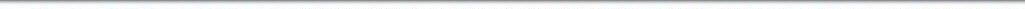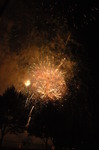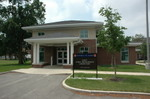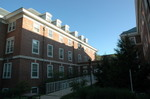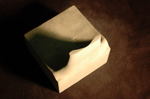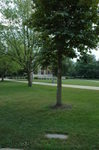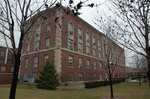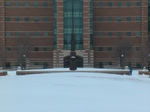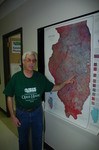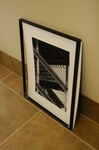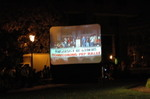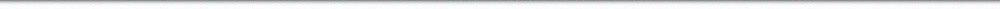N A V I G A T I O N D I G I T A L L I B R A R Y

## Repository: UIHistories Project: Book - Overview of University of Illinois for Chicago Alumni (1926) [PAGE 40]

Caption: Book - Overview of University of Illinois for Chicago Alumni (1926)
This is a reduced-resolution page image for fast online browsing.

< Previous Page [Displaying Page 40 of 63] Next Page >
[VIEW ALL PAGE THUMBNAILS]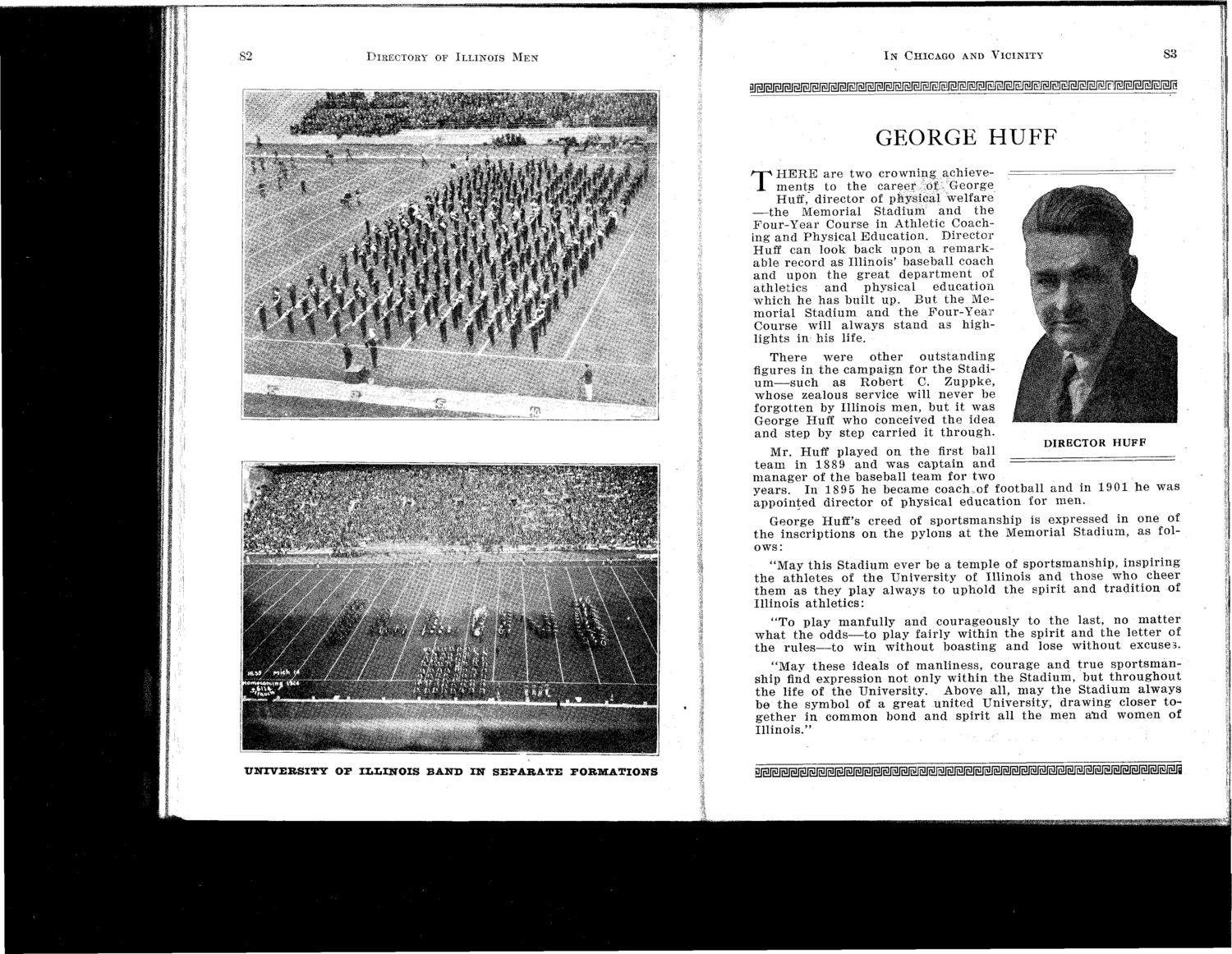### EXTRACTED TEXT FROM PAGE:

yr

82

DIRECTORY OF ILLINOIS M E N I N CHICAGO AND VICINITY S3

gjgigiigigi^^

GEORGE H U F F

T

H E R E a r e two c r o w n i n g achievem e n t s to t h e c a r e e r of George Huff, d i r e c t o r of physical welfare — t h e Memorial Stadium and the F o u r - Y e a r Course in A t h l e t i c Coaching a n d P h y s i c a l E d u c a t i o n . D i r e c t o r Huff can look back u p o n a r e m a r k able r e c o r d as Illinois' baseball coach a n d u p o n t h e g r e a t d e p a r t m e n t of athletics and physical education w h i c h he h a s built up. B u t t h e Memorial Stadium and the Four-Year C o u r s e will a l w a y s s t a n d as h i g h l i g h t s in his life. There were other outstanding figures in t h e c a m p a i g n for t h e S t a d i u m — s u c h as R o b e r t C. Z u p p k e , w h o s e zealous service will n e v e r be f o r g o t t e n by Illinois m e n , b u t it w a s George Huff who conceived t h e idea a n d s t e p by step c a r r i e d it t h r o u g h . Mr. Huff played on t h e first ball t e a m in 1889 a n d w a s c a p t a i n a n d m a n a g e r of t h e baseball t e a m for two y e a r s . In 1895 h e became coacluof football a n d in 1 9 0 1 h e w a s a p p o i n t e d d i r e c t o r of p h y s i c a l e d u c a t i o n for m e n . G e o r g e Huff's creed of s p o r t s m a n s h i p is expressed in one of t h e i n s c r i p t i o n s on t h e pylons a t t h e M e m o r i a l S t a d i u m , as folows: " M a y t h i s S t a d i u m ever be a t e m p l e of s p o r t s m a n s h i p , i n s p i r i n g t h e a t h l e t e s of t h e U n i v e r s i t y of Illinois a n d t h o s e w h o c h e e r t h e m as t h e y play a l w a y s to u p h o l d t h e spirit a n d t r a d i t i o n of Illinois a t h l e t i c s : " T o p l a y m a n f u l l y a n d c o u r a g e o u s l y to t h e last, no m a t t e r w h a t t h e o d d s — t o play fairly w i t h i n t h e s p i r i t a n d t h e l e t t e r of t h e r u l e s — t o w i n w i t h o u t b o a s t i n g a n d lose w i t h o u t excuses. " M a y t h e s e ideals of m a n l i n e s s , c o u r a g e a n d t r u e s p o r t s m a n ship find expression n o t only w i t h i n t h e S t a d i u m , b u t t h r o u g h o u t t h e life of t h e University. Above all, m a y t h e S t a d i u m a l w a y s be t h e symbol of a g r e a t u n i t e d U n i v e r s i t y , d r a w i n g closer t o g e t h e r in c o m m o n bond a n d s p i r i t all t h e m e n a n d w o m e n of Illinois."

DIRECTOR HUFF

UNIVERSITY

O F IZ»X.XNOIS B A N D I N S E P A R A T E

FORMATIONS

£ISI2J3JEJMMSJ3JSI2JS2^^